## Indicators

•Cited by SciELO
•Access statistics

•Cited by Google
•Similars in SciELO
•Similars in Google

## Print version ISSN 1692-3324On-line version ISSN 2248-4094

### Rev. ing. univ. Medellín vol.10 no.19 Medellín July/Dec. 2011

ARTÍCULOS

NUMERICAL SOLUTION OF AN EIGENVALUE PROBLEM FOR BOUNDED PLASMA

SOLUCIÓN NUMÉRICA DE UN PROBLEMA DE VALORES PROPIOS PARA UN PLASMA ACOTADO

Jaime H. Hoyos*; Carlos A. Rodríguez**

* Ph. D. in Physics, Universidad de Chile, Full time professor, Departamento de Ciencias Básicas, Grupo de investigación SUMMA, Universidad de Medellín, Medellín, Colombia. Telefax: (094)3405442. Email: jhhoyos@udem.edu.co

** Physicist, Universidad de Antioquia, Full time professor, Departamento de Ciencias Básicas, Grupo de investigación SUMMA, Universidad de Medellín, Medellín, Colombia. Telefax: (094)3405442. Email: carodriguez@udem.edu.co

Recibido: 10/02/2010

ABSTRACT

This article is the result of a research on the propagation of electromagnetic waves in a plasma found confined in a cylindrical metal structure through an intense axial magnetic field. The eigenvalue problem resulting from this study is numerically solved and frequencies of the first electromagnetic modes are obtained. Results of this work could be applied to characterize plasmas at the laboratory.

Key words: Plasma Physics, electromagnetic waves, eigenvalue problems.

RESUMEN

En este artículo investigamos la propagación de ondas electromagnéticas en un plasma que se encuentra confinado en una estructura metálica cilíndrica a través de un intenso campo magnético axial. Resolvemos numéricamente el problema de valores propios que proviene de este estudio y obtenemos las frecuencias de los primeros modos electromagnéticos. Los resultados de este trabajo podrían tener aplicaciones en caracterización de plasmas a nivel de laboratorio.

Palabras clave: física del plasma –ondas electromagnéticas– problemas de valores propios

INTRODUCTION

The theoretical study of the propagation of electromagnetic waves in a Plasma system is one of the most fundamental problems in Plasma Physics . This study requires the knowledge of the macroscopic response of the plasma to the presence of electromagnetic fields . This information is contained in the structure of physical quantities such as the dielectric function, the electrical conductivity and the current density. The theoretical derivation of these macroscopic quantities is based in kinetic microscopic models of the plasma matter which provide this information through statistical averages of the corresponding microscopic quantities.

With the knowledge of the macroscopic response, the electromagnetic boundary conditions and under an assumption of the electromagnetic fields structure is possible obtain an eigenvalue problem for the system. The solution of this problem gives the natural frequencies (eigenvalues) with the corresponding wavenumbers of the electromagnetic waves (eigenvectors or eigenmodes) propagating in the system. From the behavior of the eigenfrequencies as a function of the wavenumber (dispersion relation) is possible to calculate important quantities such as the phase and group electromagnetic wave velocities. These quantities allow to calculate the speed of the electromagnetic energy propagation through the system . In addition from the dispersion relation is possible obtain relevant information of the plasma such as the temperature and the density.

The laboratory plasmas are generally confined by strong magnetic fields inside metallic structures . Therefore, the solution of the eigenvalue problem in this case involves the solution of partial differential equations for the electromagnetic fields which must satisfy the boundary conditions. In this paper we concentrate in the study of the propagation of electromagnetic waves in a fully ionized plasma, which is confined inside a metallic cylindrical structure through a strong axial magnetic field?[5-9]. This kind of studies can be useful as a plasmas characterization tool. The reason for the latter is that the dispersion relation depends on relevant macroscopic quantities such as the plasma temperature and the plasma density profile . Several works have studied the dispersion relation of bounded plasmas for different conditions. In Ref.  the authors calculate the dispersion relation of a toroidal waveguide in the presence of a plasma column. In this case, the solution of the equations uses an analytical approximation method where the curvature of the axis of the waveguide is considered as a disturbance parameter to the straight circular cylinder approximation. Thus, the perturbed equations lead to the dispersion relation. On the other hand in Ref  the dispersion characteristics of electrostatic modes in a longitudinally magnetized parallel plate waveguide completely filled with a transversely inhomogeneous cold collisionless electron plasma are examined. In Ref.  the electrostatic hybrid resonance oscillation modes are investigated in semi-bounded magnetized dusty plasmas. There, it is found that the frequencies for the electrostatic upper- and lower-hybrid resonance oscillations are independent of the orientation of the applied magnetic field. In addition, the hybrid resonance frequencies are found to decrease as we increase the ratio of the electron plasma frequency to the electron cyclotron frequency. In Ref.  the dispersion relation for helicon waves in a uniform bounded plasma is derived by including the finite electron mass. The eigenmodes are identified and the study is related with the application of helicon waves for the generation and heating of plasmas. In Ref.  by using a quantum hydrodynamic model for bounded three-component quantum plasmas the dispersion relation for the bounded wave in quantum electron-ion-dust plasmas is obtained by carrying out a normal mode analysis. In Ref.  the effects of electromagnetic plane waves obliquely incident on a warm bounded plasma slab of finite length are studied by solving the coupled Vlasov-Maxwell set of equations. In Ref.  dust ion-acoustic surface waves propagating on the interface between a vacuum and a semi-bounded Lorentzian (kappa) plasma are kinetically investigated. In Ref.  the propagation of the TE-surface waves on a semibounded quantum plasma is investigated by using the system of generalized quantum hydrodynamic (QHD) model and Maxwell's equations.

Taking into account this context, the main body of the paper is organized as follows. In Sec. 2 we explain the basis of the physical model and establish the corresponding eigenvalue problem to study the propagation of the electromagnetic waves. In Sec. 3 we show and discuss the numerical results of this work. Finally, we give the main conclusions of our research.

1. PHYSICAL DESCRIPTION, BASIC EQUATIONS AND EIGENVALUE PROBLEM

In this section we describe the physical system and the main assumptions of our model and show the basic equations which leads to the eigenvalue problem which will be solved in the next section. The physical system is a fully ionized plasma composed by electrons and ions inside of an axially infinite metallic cylinder. This plasma is confined in a cylinder of radius a through a strong axial magnetic field. We consider the plasma to be radially inhomogeneous but axially symmetric [13-17]. We consider that the magnitude of the field is such that the cyclotron radius of the particles is much less than the cylinder radius. Therefore, we neglect the transverse motion and restrict the particles motion to the axial direction z. In this case the dynamics of particles of specie α can be described by a distribution function which is one-dimensional in the velocity space of the form: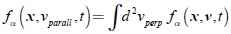where v = vparallêz + vperp is the tridimensional particle velocity with vparall the parallel speed to the magnetic field direction z and vperp the perpendicular velocity to this direction.., In order to simplify our notation in what follows we set vparallΞ v.

Due to the mass differences between the electrons and ions we consider a range of frequencies that allows neglect the response of the ions and consider only the dielectric response of the electrons. Thus, the role of the ions is neutralizing the total charge of the system. Taking into account all the previous considerations we obtain the linearized Vlasov-Maxwell system of equations which describe the dynamics of this system :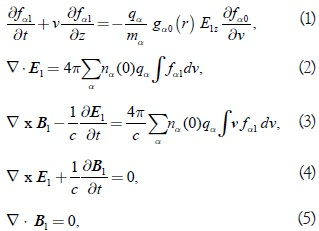where qa is the electric charge of particles of specie α = electrons, ions, fα = fα 0 + fα1 is the corresponding distribution function with fα1 << fα0 a small perturbation around the equilibrium distribution function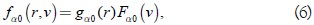with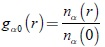giving the equilibrium radial plasma number density profile nα(r) for particles of specie α relative to the number density evaluated at the center of the cylinder nα(0). If the plasma is distributed homogeneously in the cylinder gα0(r) = 1. otherwise gα0(r) is an arbitrary function of the radius r. On the other hand Fα0(v) is the Maxwell-Boltzman distribution function for the axial particle velocities.

In Eqs. (1-5) the electromagnetic fields have been linearized as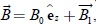with B0 a strong axial equilibrium magnetic field and the electric field as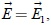where B1 <<, and E1 are small perturbations. The strong axial magnetic field promotes that the electrons to move preferentially along the cylinder axis, thus, the Transverse electric modes (TE) which have the electric field confined to the transversal plane are not modified by the plasma presence respect to that structure in a vacuum cylinder. Thus, we restrict our study only to the Transverse Magnetic modes (TM) which are modified by the plasma presence. From the structure of the Eqs. (1-5) is possible to show that all the components of the electromagnetic field in a TM mode can be obtained from the knowledge of only the axial electric field component . Thus, and according to the cylindrical geometry of the system we expand the axial electric field as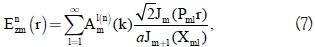where Aml(n)(k) are the expansion coefficients which define the electric field structure for the transverse magnetic mode TMmn with the indices m,n being the azimutal and radial number respectively. Jm(X) are the Bessel functions of first specie and order m, and Pml is the radial wavenumber which is obtained from the boundary condition Jm(Pmla) = 0 (perfect conductor at the cylinder walls ), thus Pmla = Xml and Xml are the zeros of the equation Jm(X) = 0. Introducing the above expansion in the Eqs. (1-5) and considering harmonic propagating fields with angular frequency ω and axial wavenumber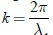with λ the wavelength, we obtain the eigenvalue problem for this system: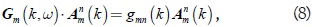where gmn(k) are the eigenvalues, Amn(k) the corresponding eigenvectors and the matrix Gm(k,ω) is defined in terms of the auxiliar matrix Qm(k,ω)–gmn(k)1. Thus, for each k it is neccessary to find the eigenfrequencies ωmn(k) that satisfy the Eq. (8). The elements of the matrix Qm(k,ω) are given by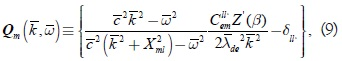where we have introduced the dimensionless variables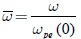the angular frequency normalized by the electrons plasma frequency evaluated at the center of the cylinder,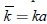the wavenumber normalized by the cylinder radius,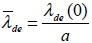the Debye length for the electrons which depends on the plasma temperture ,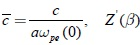is the first derivative of the Plasma dispersion function ,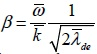and the eigenvalues which contain the eigenfrequencies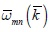are given by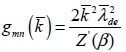.

2. NUMERICAL RESULTS

In this section we calculate numerically the eigenfrequencies of the first three modes given by Eq.(8). To achieve this goal we use the numerical method developed by Serizawa . The basis of this method are explained in Appendix. We focus our numerical analysis in the symmetrical modes, i.e, TM01, TM02, TM03. We use the inhomogeneuous radial density profile for the electrons as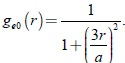. This inhomogeneous density profile adjusts well the profile measured in the classic experiment of Malmberg and Wharton  in which they studied experimentally the propagation of waves in a long cylinder with a confined plasma on it. The numerical calculations were done with the parameters of the Malmberg and Wharton experiment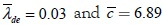and we used for the solution of Eq. (9) the numerical methods developed in Refs. [21-22]. For our calculations we selected the size of the matrix Eq.(9) as 15x15. We found numerical differences with the eigenfrequencies obtained with the matrix 14x14 of order 10–5.

For each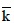we found the presence of two modal branches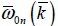. One of them is a low frequency mode (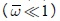) while the other one is a high frequency mode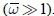In Fig. 1 we show the numerical results for the first three low and high frequency modes (full line) and we compare the results with those modes arising from a homogeneous plasma, i.e. ge0(r) = 1 (dashed line). We observe from this figure that the low-frequency modes for the inhomogeneous case (full line) are lower than the corresponding ones for the homogeneous case (dashed line). This can be explained taking account that in the inhomogeneous case the effective density across the cylinder decreases respect to the homogeneous case which completely fills the entire cylinder. In addition for small wave-lengths compared with the cylinder radius (ka >> 1) the plasma ignores the boundaries, thus, the behavior must correspond to a not bounded plasma. It is well known that for not bounded plasma case the frequencies of the low frequency waves (electrostatic waves) are proportional to the plasma density [1, 5].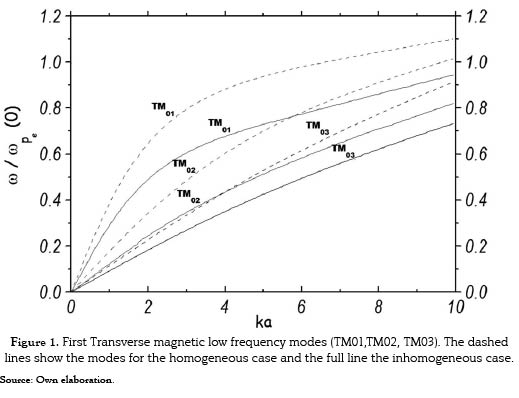On the other hand, in Fig. 2 we compare the TM01 low-frequency mode for the inhomogeneous case (full line) with the corresponding results obtained in the Malmberg and Wharton experiment (circles) and we observe a good agreement between the two curves. This is a strong verification of our numerical method and in general of our physical model.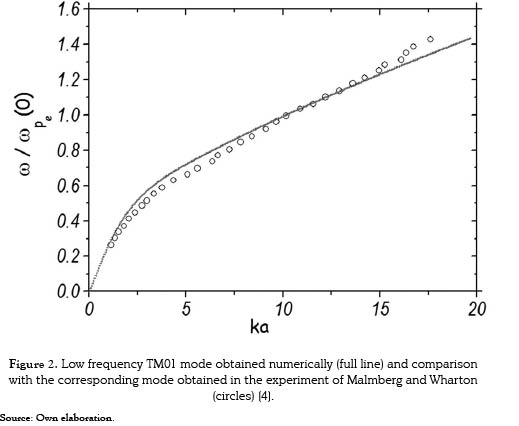In Figs 3-4 we show the same modes than before but now for the high frequency electromagnetic waves. We observe that in all the cases the presence of a cutoff frequency in the limit ka → 0. For these modes, the waves with frequencies lower than this cutoff are forbidden. In addition in Fig. 3 we observe that for high frequencies the phase velocity () of the waves tends to the light speed at vacuum. This fact is expected since in this regime of frequencies the waves ignores the plasma presence.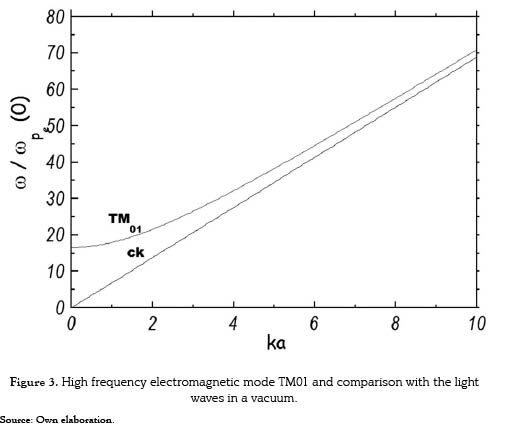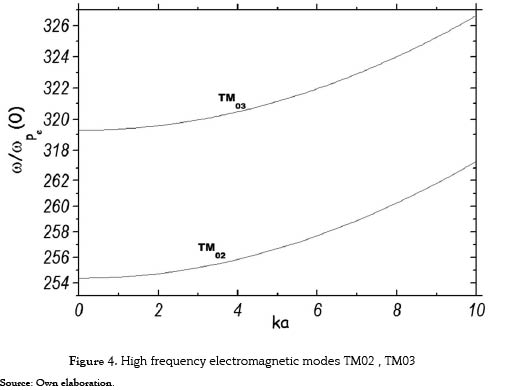3 SUMMARY AND CONCLUSIONS

We found numerically the eigenfrequencies of the first symmetrical transverse magnetic modes TM0n modes in a radially inhomogeneous plasma confined in a cylinder through a strong magnetic field. To describe the dynamics of the system we used the Vlasov-Maxwell system of equations. We expanded the electromagnetic fields in an orthonormal basis which lead to find the eigenvalues of a matrix whose elements depend on the radial density profile, the plasma temperature and the cylinder radius. We solved numerically this eigenvalue problem and we found the presence of two main modal branches. One of these branches is a low-frequency mode with frequencies much lower than the natural plasma frequency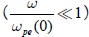and the high frequency mode corresponds to the opposite regime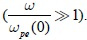We found that for the high frequency mode the phase speed of the waves tends to the light speed velocity at vacuum. This is reasonable since for this high frequency range the waves ignores the presence of the plasma. We found that for the low frequency mode the plasma inhomogeneity produces a decrease of the frequencies respect to the homogeneous case. This can be explained taking account that for small wavelengths compared with the cylinder radius (ka >> 1) the plasma ignores the boundaries, thus, the behavior must correspond to an infinite plasma medium on which the frequencies of the low frequency waves (electrostatic waves) are proportional to the plasma density. In addition, we compared the numerical results of the first electrostatic mode with experimental measurements and we found a good agreement. This comparison gives validity to our numerical methods and also to the physical model and assumptions. The elements of the matrix Eq. (9) depend on the density an plasma temperature through the electron Debye length. Thus, an experimental measurement of the frequencies of the propagating waves as was done by Malmberg and Wharton can be used as a characterization tool . A precise information of parameters as the temperature and density can be crucial to improve the efficiency of plasma assisted processes which are used in materials technology, for instance, in material hard coating processes.

ACKNOWLEDGMENTS

JH thanks Alfonso Devia and Carlos Diaz for useful physical discussions around this research. JH thanks the hospitality of Laboratorio de Física del Plasma at Universidad Nacional de Colombia, Sede Manizales. This manuscript was elaborated as part of the research shock plan of the University of Medellín 2009-2010 and the research project ''Campos Magnéticos'' (University of Medellin project Identification Number: 530).

REFERENCES

 N. A. Krall, y A. W. Trivelpiece, Principles of Plasma Physics, New York: McGraw-Hill, Inc, 1973, 674 p.         [ Links ]

 S. Ichimaru, Basic Principles of Plasma Physics: A Statistical Approach, Massachusetts: Bejamin Reading, Inc, 1973, 324 p.         [ Links ]

 V. L. Ginzburg, The propagation of Electromagnetic Waves in Plasmas, 2 ed., Oxford, New York: Pergamon Press, 1970, 615 p.         [ Links ]

 J. H. Malmberg, y C. B. Wharton, ''Dispersion of Electron Plasma Waves,'' Phys.Rev.Letts, vol. 17, no. 4, pp. 175-178, 1966.         [ Links ]

 F. M. Aghamir et al., ''Dispersion Characteristics of a Plasma Column in a Toroidal Waveguide,'' IEEE Transactions on Plasma Science, vol. 39, no. 5, pp. 1204 - 1212, 2010.         [ Links ]

 P. d. Santis, y G. Franceschetti, ''Dynamic Modes in Open Gyromagnetic Waveguides,'' Journal of Applied Physics, vol. 43, no. 4, pp. 513-515, 1973.         [ Links ]

 C.-G. Kim, y Y.-D. Jung, ''Electrostatic hybrid resonance modes in semi-bounded magnetized dusty plasmas '' Journal of Physics D: Applied Physics, vol. 39, no. 1, pp. 119, 2006.         [ Links ]

 M. Shoucri, ''Helicon waves in a cylindrical plasma,'' Canadian Journal of Physics, vol. 77, no. 5, pp. 385-391, 1999.         [ Links ]

 K.-Z. Zhang, y J.-K. Xue, ''Streaming instability in bounded three-component quantum plasmas,'' Phys. Plasmas, vol. 17, no. 3, 2010.         [ Links ]

 J. R. Angus et al., ''Kinetic theory of electromagnetic plane wave obliquely incident on bounded plasma slab,'' Phys. Plasmas, vol. 17, no. 10, 2010.         [ Links ]

 T-Kim, y M.-J. Lee, ''Dust ion-acoustic surface waves and Landau damping in a semi-bounded Lorentzian plasma,'' Phys. Plasmas, vol. 15, no. 12, 2008.         [ Links ]

 B. F. Mohamed, y M. A. Aziz, ''Propagation of TE-Surface Waves on Semi-Bounded Quantum Plasma,'' International Journal of Plasma Science and Engineering, [En línea], vol. 2010, no., Disponible: http://www.hindawi.com/archive/2010/693049/cta/, 2010.         [ Links ]

 C. J. Diaz, ''On the Vlasov-Poisson system of equations for an inhomogeneous cylindrical plasma '' Plasma Phys., vol. 23, no. 5, pp. 455-472, 1981.         [ Links ]

 A. Devia, ''Ondas Electromagnéticas en Plasmas cilíndricos e Inhomogéneos,'' tesis de Tesis de maestría, Universidad del Valle, 1983.         [ Links ]

 A. Devia et al., ''Electromagnetic oscilations in cylindrical plasmas with electron beams interactions,'' Astrophysics and Space Science, vol. 256, pp. 321-326, 1997.         [ Links ]

 J. Hoyos, ''Relación de dispersión para ondas electromagnéticas en un plasma cilíndricos,'' tesis de pregrado en Física, Universidad Nacional de Colombia, 2001.         [ Links ]

 J. Hoyos et al., ''Transverse Magnetic Modes in a strongly magnetized bounded inhomogeneous plasma,'' presentado en Simposio Chileno de Física, Concepción, 2002.         [ Links ]

 A. Vlasov, ''On the Kinetic Theory of an Assembly of Particles with Collective Interaction,'' Journal of Physics USSR, vol. 9, no. 1, pp. 25-40, 1945.         [ Links ]

 J. D. Jackson, Electrodinámica Clásica, 2 ed., Madrid: Editorial Alhambra, 1980, 861 p.         [ Links ]

 B. D. Fried, y S. D. Conte, The Plasma Dispersion Function, New York: Academic Press, 1961, 419 p.         [ Links ]

 T.Watanabe, y H. Daigaku, Efficient computation of the plasma dispersion function Z, Hiroshima: Institute for Fusion Theory, Hiroshima University, 1979, 14 p.         [ Links ]

 Y. Serizawa et al., ''A Numerical Method for Eigenvalue Problems of Integral Equations,'' J. Phys. Soc. Jpn, vol. 52, pp. 28-35, 1983.         [ Links ]

 G. Tribulato, ''Characterization of a magnetized plasma in cylindrical geometry,'' tesis de maestría en Física, Universidad de Tromso, Noruega, 2007.         [ Links ]

APPENDIX. NUMERICAL METHOD

In this work we solve the eigenvalue problem given by Eq. (9) which can be written as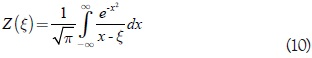For each k we need to find the corresponding ω (k), these values must satisfy the above equation. Thus, we solve the generic martricial system: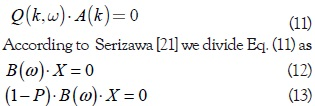where P is a proyection operator of N grade

To obtain ω and X we make a Taylor expansion around given values ω0 and X0 as follows

ω = ω0 + ω1 (14)

X = X0 + X1 (15)

The above procedure leads to a matrix system of equations that we solve for ω and X.

On the other hand, the eigenvalue problem of Eq. (9) requires the knowledge of the Plasma dispersion Function . This function is defined by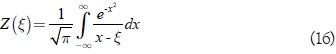for Im(ξ) > 0 and can be defined for Im(ξ) < 0 by analytic continuation. Some useful relations are: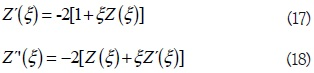The numerical calculation of Z(ξ) is based on Ref.(21) where the residue theorem of the complex variable theory is used.All the contents of this journal, except where otherwise noted, is licensed under a Creative Commons Attribution License